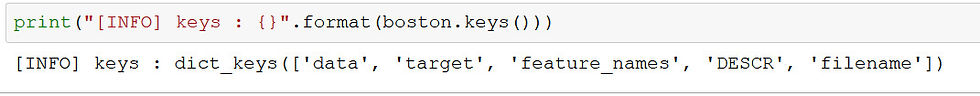top of page
Search

# Predict Boston House Prices Using Python & Linear Regression

In machine learning, the ability of a model to predict continuous or real values based on a training dataset is called Regression. With a small dataset and some great python libraries, we can solve such a problem with ease.

In this blog post, we will learn how to solve a supervised regression problem using the famous Boston housing price dataset. Other than location and square footage, a house value is determined by various other factors. Let’s analyze this problem in detail and using machine learning model to predict a housing price.

Dependencies

• pandas - To work with solid data-structures, n-dimensional matrices and perform exploratory data analysis.

• matplotlib - To visualize data using 2D plots.

• seaborn - To make 2D plots look pretty and readable.

• scikit-learn - To create machine learning models easily and make predictions.

Boston Housing Prices Dataset

In this dataset, each row describes a boston town. There are 506 rows and 13 attributes (features) with a target column (price).

The problem that we are going to solve here is that given a set of features that describe a house in Boston, our machine learning model must predict the house price. To train our machine learning model with boston housing data, we will be using scikit- learn’s boston dataset.

We will use pandas and scikit-learn to load and explore the dataset. The dataset can easily be loaded from scikit-learn datasets module using load_boston function

```import pandas as pd
from sklearn import datasets

There are four keys in this dataset using which we can access more information about the dataset .["data ", "target", "feature_name" and "DESCR"] are the four keys which could be accessed using keys() on the dataset variable.To know the description of each column name in this dataset, we can use DESCR to display the description of this dataset .Exploratory Data Analysis

We can easily convert the dataset into a pandas dataframe to perform exploratory data analysis. Simply pass in the boston.data as an argument to pd.DataFrame(). We can view the first 5 rows in the dataset using head() function.

```bos = pd.DataFrame(boston.data, columns = boston.feature_names)
bos['PRICE'] = boston.targetExploratory Data Analysis is a very important step before training the model. Here, we will use visualizations to understand the relationship of the target variable with other features.

Let’s first plot the distribution of the target variable. We will use the histogram plot function from the matplotlib library.

```sns.set(rc={'figure.figsize':(11.7,8.27)})
plt.hist(bos['PRICE'],color ="brown", bins=30)
plt.xlabel("House prices in \$1000")
plt.show()```

histogram plotWe can see from the plot that the values of PRICE are distributed normally with few outliers. Most of the house are around 20–24 range (in \$1000 scale)

Now, we create a correlation matrix that measures the linear relationships between the variables. The correlation matrix can be formed by using the corr function from the pandas dataframe library. We will use the heatmap function from the seaborn library to plot the correlation matrix.

```#Created a dataframe without the price col, since we need to see the #correlation between the variables
bos_1=pd.DataFrame(boston.data, columns=boston.feature_names)

correlation_matrix=bos_1.corr().round(2)
sns.heatmap(data=correlation_matrix, annot=True)```The correlation coefficient ranges from -1 to 1. If the value is close to 1, it means that there is a strong positive correlation between the two variables. When it is close to -1, the variables have a strong negative correlation.

By looking at the correlation matrix we can see that RM has a strong positive correlation with PRICE (0.7) where as LSTAThas a high negative correlation with PRICE (-0.74).

```plt.figure(figsize=(20, 5))

features = ['LSTAT', 'RM']
target = bos['PRICE']

for i, col in enumerate(features):
plt.subplot(1, len(features) , i+1)
x = bos[col]
y = target
plt.scatter(x, y,color='green', marker='o')
plt.title("Variation in House prices")
plt.xlabel(col)
plt.ylabel('"House prices in \$1000"')

```The prices increase as the value of RM increases linearly. There are few outliers and the data seems to be capped at 50.

The prices tend to decrease with an increase in LSTAT. Though it doesn’t look to be following exactly a linear line.

‘RM’ shows positive correlation with the House Prices we will use this variable.

```X_rooms = bos.RM
y_price = bos.PRICE

X_rooms = np.array(X_rooms).reshape(-1,1)
y_price = np.array(y_price).reshape(-1,1)```

Since we need to test our model, we split the data into training and testing sets. We train the model with 80% of the samples and test with the remaining 20%. We do this to assess the model’s performance on unseen data.

To split the data we use train_test_split function provided by scikit-learn library. We finally print the shapes of our training and test set to verify if the splitting has occurred properly.

Splitting dataset into training and testing

Since we need to test our model, we split the data into training and testing sets. We train the model with 80% of the samples and test with the remaining 20%. We do this to assess the model’s performance on unseen data.

To split the data we use train_test_split function provided by scikit-learn library. We finally print the shapes of our training and test set to verify if the splitting has occurred properly.

`X_train_1, X_test_1, Y_train_1, Y_test_1=train_test_split(X_rooms, y_price, test_size=0.2, random_state=5)`

Training and Testing the Model

Here we use scikit-learn’s LinearRegression to train our model on both the training and check it on the test sets. and check the model performance on the train dataset.

```reg_1=LinearRegression()
reg_1.fit(X_train_1, Y_train_1)

y_train_predict_1=reg_1.predict(X_train_1)
rmse= (np.sqrt(mean_squared_error(Y_train_1, y_train_predict_1)))
r2=round(reg_1.score(X_train_1, Y_train_1),2)

print('RMSE is {}'.format(rmse))
print('R2 score is {}'.format(r2))
print("\n")```Model Performance

The root-mean-square deviation (RMSD) or root-mean-square error (RMSE) is a frequently used measure of the differences between values (sample or population values) predicted by a model or an estimator and the values observed.

```y_pred_1=reg_1.predict(X_test_1)
rmse= (np.sqrt(mean_squared_error(Y_test_1, y_pred_1)))
r2=round(reg_1.score(X_test_1, Y_test_1),2)

print("Root Mean Squared Error: {}".format(rmse))
print("R^2: {}".format(r2))
print("\n")```Plotting the Model

plotting scatter plot for our model performance which x-axis label = features of house and y-axis label = price of house

```prediction_space = np.linspace(min(X_rooms), max(X_rooms)).reshape(-1,1)
plt.scatter(X_rooms,y_price)
plt.plot(prediction_space, reg_1.predict(prediction_space), color = 'black', linewidth = 3)
plt.ylabel('value of house/1000(\$)')
plt.xlabel('number of rooms')
plt.show()```